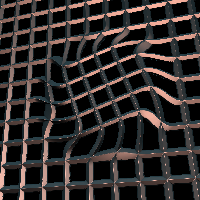# magnet

Pulls or twists space within an area.

If the magnet definition input is connected, that operator is used to determine how much transformation to apply at each point in space. If there is no magnet definition connected, the magnet is based around a center point with a radius, and a blending region.

## Parameters

• `Enable`:
• `Amount`: How much effect to apply overall.
• `Center`: The center position of the magnet (used if the magnet definition is not connected).
• `Radius`: The radius of the magnet area.
• `Fade`: The width of the blending region between the magnet and the rest of space.
• `Scale`: Scaling to be applied within the magnet area.
• `Rotate`: Rotation to be applied within the magnet area.

## Inputs

• `definition_in`: (required)
• Coordinate Types: `float` `vec2` `vec3`
• Context Types: `Context` `MaterialContext` `CameraContext` `LightContext` `RayContext` `ParticleContext`
• Return Types: `float` `vec4` `Sdf` `Ray` `Light` `Particle`
• `Magnet`: (optional) Magnet definition used to determine how much transformation to apply at each point. If this is an operator that produces an SDF or float value, that value is used to decide how far each point is from the magnet. If it returns a vec4, it is used to determine where the magnet center position is relative to each point.
• Coordinate Types: `float` `vec2` `vec3`
• Context Types: `Context` `MaterialContext` `CameraContext` `LightContext` `RayContext` `ParticleContext`
• Return Types: `float` `vec4` `Sdf`
• `Easing`: (optional) Easing function used to control how the blending region is smoothed.
• Coordinate Types: `float`
• Context Types: `Context` `MaterialContext` `CameraContext` `LightContext` `RayContext` `ParticleContext`
• Return Types: `float`